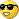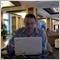# All John Ehlers Indicators...418

Hi all...

I'm dedicating this thread to all indicators by John Ehler's in the hope that we can get/make as many of them for MT4 as possible.

Please post any MT4 John Ehlers indicators

that you have here as outlined in his books "Rocket Science for Traders", "Cybernetic Analysis for Stocks and Futures", "Mesa and Trading Market Cycles".

Please post any coding for indicators that programmers could convert to Mql4.

Thanks to Igorad and others for already programming many of these indicators.

To kick things off see my attachments...

Gramski.

Files:418

More

More attachments..

Files:
mama.mq4 5 kb418

Yet more

More MT4 indicators....

Does anyone have any of the following?

RST_Hilbert_Sinewave

RST_Hilbert_Oscillator

RST_Hilbert_Phase

RST_Homodyne_Descriminator

lsm

MESA

CyberCycle

Or any hybrid type indicators?

e.g

Fisher CyberCycle

Fisher Stochastic RVI

Files:418

Some reading regarding MAMA, Laguerre RSI and Fisher Transform....

GramskiFiles:418

MT3 to MT4.

I found these Mql3 Indicators if anyone could convert it to Mql4?

RST_Hilbert_Sinewave

RST_Hilbert_Oscillator...

Gramski.

Files:Moderator
115891

I have two indicators for MT3.

I did not check it.

Files:
mesa.mql 4 kb
rs-herst.mql 2 kb418

Thanks NewDigital,

I also found these mt3 indicators...

I know that the Sinewave indicator (that I posted above) looks interesting.

There are other ones like Cybercycle and Stochastic RVI that I don't have.

If anyone has experience converting mt3 to mt4 please have a go...

Gramski.

Files:
lsm.mql 3 kb287

The Laguerre indicator is fabolous.How does the laguerre filter work? Any description?418

TheWicker:
The Laguerre indicator is fabolous.How does the laguerre filter work? Any description?

I don't use the Laguerre filter but LaguerreRSI is one of the main indicators in my system.

I have a simple alert EA for the Laguerre RSI signal with AbsoluteStrength as a filter to stop the LRSI falsing signaling all the time.

After a signal I usually check LSMA and TTM to confirm an entry and check that LRSI has 'printed'.

The only problem I can see with LaguerreRSI is that it doesn't show continuation very well...you need to use another rule for that...

Gramski.Files:11

I got one.

For mt3 indicators of RST sinewave/phase/homodyn listed above ,I have done some test and personally think they may have some errors in the programs because there is wrong performance corelative with price according to John's explanation in the book.

BRs

Stl

Files:418

Thanks,

Here is the tradestation code of sinewave indicator.

Typ : Indicator, Name : Sine Wave Indicator

Inputs:

Price((H+L)/2);

Vars:

InPhase(0),

Phase(0),

DeltaPhase(0),

count(0),

InstPeriod(0),

Period(0),

DCPhase(0),

RealPart(0),

ImagPart(0);

If CurrentBar > 5 then begin

Value1 = Price - Price;

Value2 =Value1;

Value3 =.75*(Value1 - Value1) + .25*(Value1 - Value1);

InPhase = .33*Value2 + .67*InPhase;

{Use ArcTangent to compute the current phase}

If AbsValue(InPhase +InPhase) > 0 then Phase =

{Resolve the ArcTangent ambiguity}

If InPhase 0 then Phase = 180 - Phase;

If InPhase < 0 and Quadrature < 0 then Phase = 180 + Phase;

If InPhase > 0 and Quadrature < 0 then Phase = 360 - Phase;

{Compute a differential phase, resolve phase wraparound, and limit delta phase errors}

DeltaPhase = Phase - Phase;

If Phase 270 then DeltaPhase = 360 + Phase - Phase;

If DeltaPhase < 1 then DeltaPhase = 1;

If DeltaPhase > 60 then Deltaphase = 60;

{Sum DeltaPhases to reach 360 degrees. The sum is the instantaneous period.}

InstPeriod = 0;

Value4 = 0;

For count = 0 to 40 begin

Value4 = Value4 + DeltaPhase[count];

If Value4 > 360 and InstPeriod = 0 then begin

InstPeriod = count;

end;

end;

{Resolve Instantaneous Period errors and smooth}

If InstPeriod = 0 then InstPeriod = InstPeriod;

Value5 = .25*InstPeriod + .75*Value5;

{Compute Dominant Cycle Phase, Sine of the Phase Angle, and Leadsine}

Period = IntPortion(Value5);

RealPart = 0;

ImagPart = 0;

For count = 0 To Period - 1 begin

RealPart = RealPart + Sine(360 * count / Period) * (Price[count]);

ImagPart = ImagPart + Cosine(360 * count / Period) * (Price[count]);

end;

If AbsValue(ImagPart) > 0.001 then DCPhase = Arctangent(RealPart / ImagPart);

If AbsValue(ImagPart) <= 0.001 then DCPhase = 90 * Sign(RealPart);

DCPhase = DCPhase + 90;

If ImagPart < 0 then DCPhase = DCPhase + 180;

If DCPhase > 315 then DCPhase = DCPhase - 360;

Plot1(Sine(DCPhase), "Sine");